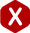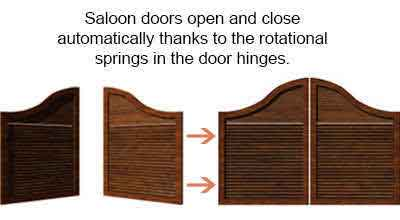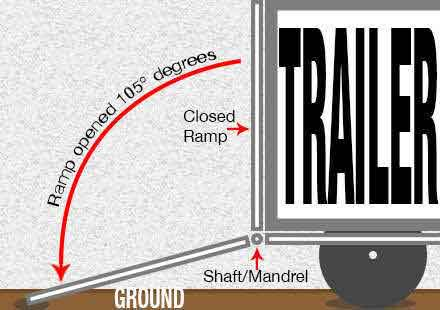<# Torsion Spring Uses

#### Torsion springs are used to exert a rotational force also known as torque. Their legs are radially impulsed to create this winding/twisting effect.Torsion springs are used in several applications which require the spring to deflect radially. Unlike compression and extension springs which perform a linear deflection. This is why the units of deflection of a torsion spring is in degrees of travel vs. being in inches or millimeters like it is for the previously mentioned spring types.

Because of how the springs are used, you may be able to find them in door hinges, hatches, lids, or even trailer ramps. Heavy duty torsion springs may be used in some of these applications but heavy duty springs can also be highly stressed thus reducing its elasticity. This means that the heavy duty torsion spring you are planning to use might not be able to give you the distance traveled you required and it could take a permanent set which would reduce its amount of torque.The solution to over stressed heavy duty torsion springs is using more than one spring to work in parallel. When two or more springs work in parallel, the force of the spring is multiplied by the number of springs working together. This is usually done for trailer ramps and door hinges. Using this method allows you to get more force with springs that aren’t under much stress so it gives you the opportunity to have more elasticity/deflection.

In the example to the right, you’re able to see a trailer ramp in use. To calculate the amount of torsion spring rate required for the ramp’s weight to be easily lifted after a certain distance traveled, you must first calculate the weight of the ramp. This is done by multiplying the length of the ramp in feet by the dead weight of the ramp in pounds. This will give you the exact weight being put on the spring. Then, you must divide the total weight of the ramp by the distance traveled as you would do in the ordinary torsion spring rate formula which asks you to divide the torque by the deflection.